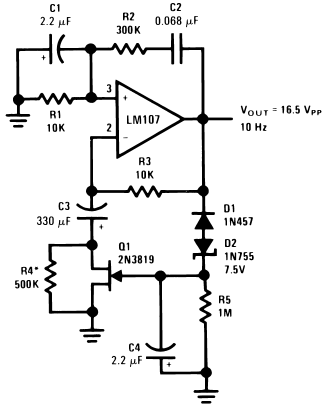## Sine Wave Oscillator

### By internum

An amplitude-stabilized sine-wave oscillator. This circuit provides high purity sine-wave output down to low frequencies with minimum circuit complexity.

An amplitude-stabilized sine-wave oscillator is shown in Figure 1. This circuit provides high purity sine-wave output down to low frequencies with minimum circuit complexity. An important advantage of this circuit is that the traditional tungsten filament lamp amplitude regulator is eliminated along with its time constant and linearity problems.Figure 1.Wien Bridge Sine Wave Oscillator

In addition, the reliability problems associated with a lamp are eliminated.

The Wien Bridge oscillator is widely used and takes advantage of the fact that the phase of the voltage across the parallel branch of a series and a parallel RC network connected in series, is the same as the phase of the applied voltage across the two networks at one particular frequency and that the phase lags with increasing frequency and leads with decreasing frequency. When this network—the Wien Bridge—is used as a positive feedback element around an amplifier, oscillation occurs at the frequency at which the phase shift is zero. Additional negative feedback is provided to set loop gain to unity at the oscillation frequency, to stabilize the frequency of oscillation, and to reduce harmonic distortion.

The circuit presented here differs from the classic usage only in the form of the negative feedback stabilization scheme. Circuit operation is as follows: negative peaks in excess of −8.25V cause D1 and D2 to conduct, charging C4. The charge stored in C4 provides bias to Q1, which determines amplifier gain. C3 is a low frequency roll-off capacitor in the feedback network and prevents offset voltage and offset current errors from being multiplied by amplifier gain.

Distortion is determined by amplifier open-loop gain and by the response time of the negative feedback loop filter, R5 and C4. A trade-off is necessary in determining amplitude stabilization time constant and oscillator distortion. R4 is chosen to adjust the negative feedback loop so that the FET is operated at a small negative gate bias. The circuit shown provides optimum values for a general purpose oscillator.796910 December 2007